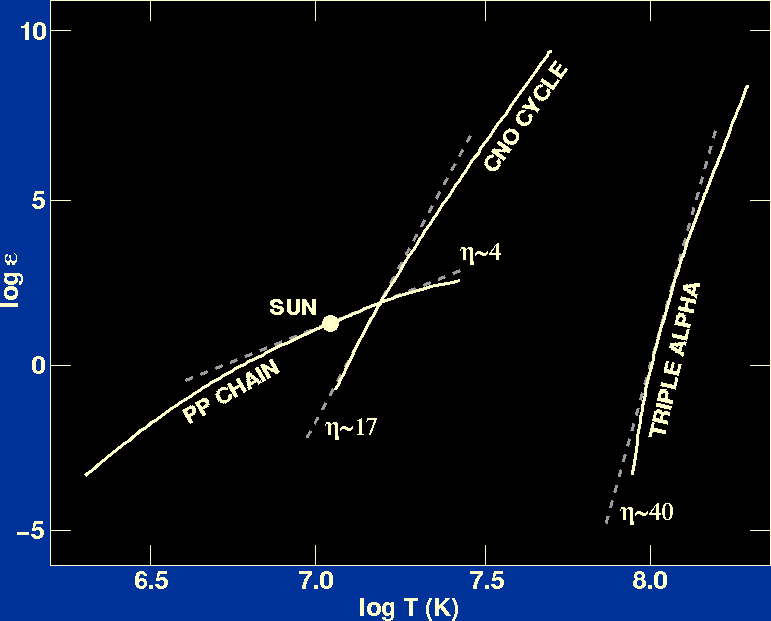We have seen that the probabililty of fusion is related to the energies of the particles involved and hence to the temperature of the plasma. Using experimental values for the rates at which the PP chain, CNO cycle and triple-alpha reactions occur, it is possible to plot the energy release of each reaction as a function of temperature, as shown in figure 20.

 Figure 20: Rate of energy release from hydrogen and helium burning as a function of temperature.indicates the gradient of the straight-line fit to the curve.The energy released by the PP chain and CNO cycle are smooth functions of temperature. In a limited temperature range we can replace the true dependence on temperature by power law fits to the curves in figure 20. These fits have the following approximate form:

 PP chain:=0T 4 CNO cycle:=0T 17 Triple-alpha reaction:=02 T 40,

whereis the energy release per unit mass per unit time,is the density of the stellar material, T is the temperature of the stellar material and0 is a constant which depends on the chemical composition of the stellar material.

The above expressions forreflect the fact that the rate of fusion is a very sensitive function of temperature, because the probability of tunnelling depends on kinetic energy, which in turn depends on temperature. Furthermore, it can be seen that fusion reactions involving successively heavier elements (in ascending order: the PP chain, the CNO cycle and the triple-alpha reaction) become even more temperature dependent, and require higher temperatures to operate, in order to overcome the larger Coulomb barrier due to the heavier (and hence more positively charged) nuclei. As well as depending on temperature,also depends on the density of the stellar material. For two-particle reactions such as PP chain and CNO cycle reactions, the dependence on density is linear, whereas for three-particle reactions such as the triple-alpha process, the dependence is quadratic.

Clearly, the true laws of energy release are not power laws, but the expressions given are good approximations as the energy release increases very rapidly with temperature and the range of temperatures in which significant release occurs is small. We shall see in the next part of the course how the use of the above approximate expressions for the laws of energy production enables us to obtain useful qualitative information about the structure of stars.